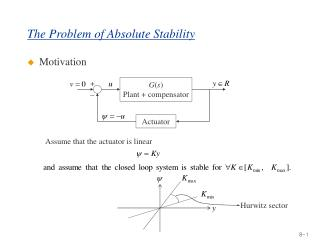DownloadDownload PresentationThe Problem of Absolute Stability

# The Problem of Absolute Stability

Download Presentation## The Problem of Absolute Stability

- - - - - - - - - - - - - - - - - - - - - - - - - - - E N D - - - - - - - - - - - - - - - - - - - - - - - - - - -
##### Presentation Transcript

1. + _ G(s) Plant + compensator Actuator The Problem of Absolute Stability • Motivation Assume that the actuator is linear Hurwitz sector

2. Aizerman conjecture Assume now that the actuator is nonlinear, for instance, a saturator This question was posed by M.A. Aizerman in 1940’s. Aizerman conjecture :

3. Kalman conjecture Kalman conjecture : A answer was first proposed by A.I. Lurie. Popov, Kalman, Yakubovich and others contributed to the solution. Sometimes this problem is called the Lurie problem. N.B :

4. Problem Formulation Plant Assumptions

5. Problem (Continued) We can rewrite the sector condition  as

6. Problem (Continued) Consider the decentralized feedback Define and Then p-dim sector condition is symmetric positive definite diagonal matrix

7. Def : Problem (Continued) - Generalization for centralized case Introduce Then where again

8. Notation Define : Problem (Continued) Consider again

9. Remarks Remarks • Absolute stability  not another type of stability • Absolute stability gives a measure of robustness • No constructive necessary and sufficient conditions have beenfound as yet. The main tool is the Kalman-Yakubovich-Popov Lemma.

10. Solution • Approach to the solution To find out conditions of absolute stability Find a Lyapunov function good for a continuum of systems – all with nonlinearities in the sector  Two types of Lyapunov functions are typically used. Here the conditions are less conservative (Popov Criterion).

11. Solution (Continued)

12. Kalman – Yakubovich-Popov Lemma

13. Circle Criterion • Circle Criterion

14. K – Y – P Lemma

15. K – Y – P Lemma (Continued) Lemma :

16. 0 G(s) Generalization To eliminate the restriction on A to be Hurwitz  loop transformation (pole shifting) G(s)

17. Generalization (Continued) Obviously

18. Generalization : Case 1

19. Generalization : Case 1

20. Generalization : Case 1   

21. Generalization : Case 1

22. Generalization : Case 2

23. Generalization : Case 3

24. Summarizing

25. Summarizing

26. Popov Criterion • Popov Criterion Popov

27. Popov Criterion (Continued)

28. Popov Criterion (Continued)

29. Popov Criterion (Continued)

30. Popov Criterion (Continued)

31. Popov Criterion (Continued)

32. Theorem Theorem :

33. Theorem(Continued)

34. K can be as large as possible Finite sector Theorem(Continued) Graphically

35. Theorem(Continued)

36. Theorem(Continued)